# pytorch中文文档-torch.nn常用函数-待添加

https://pytorch.org/docs/stable/nn.html

1）卷积层

### class torch.nn.Conv2d(in_channels, out_channels, kernel_size, stride=1, padding=0, dilation=1, groups=1, bias=True)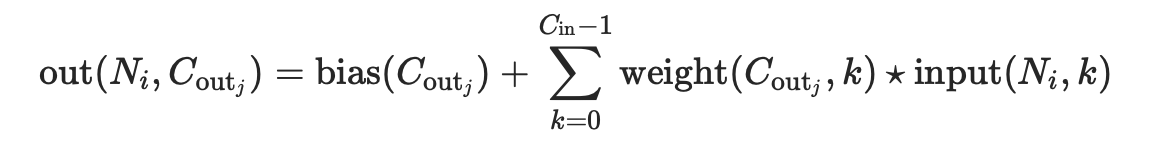• stride: 控制相关系数的计算步长
• dilation: 用于控制内核点之间的距离；
• groups: 控制输入和输出之间的连接：可见pytorch的函数中的group参数的作用
1. group=1，输出是所有的输入的卷积；
2. group=2，此时相当于有并排的两个卷积层，每个卷积层计算输入通道的一半，并且产生的输出是输出通道的一半，随后将这两个输出连接起来得到结果；
3. group=in_channels,每一个输入通道和它对应的卷积核进行卷积，该对应的卷积核大小为• 参数kernel_sizestride,paddingdilation：
1. 也可以是一个int的数据，此时卷积height和width值相同;
2. 也可以是一个tuple数组，tuple的第一维度表示height的数值，tuple的第二维度表示width的数值，当是数组时，计算时height使用索引为0的值，width使用索引为1的值

Parameters：

• in_channels(int) – 输入信号的通道
• out_channels(int) – 卷积产生的通道
• kerner_size(int or tuple) - 卷积核的尺寸
• stride(int or tuple, optional) - 卷积步长，默认为1
• padding(int or tuple, optional) - 输入的每一条边补充0的层数，默认为0
• dilation(int or tuple, optional) – 卷积核元素之间的间距,默认为1
• groups(int, optional) – 从输入通道到输出通道的阻塞连接数。默认为1
• bias(bool, optional) - 如果bias=True，添加可学习的偏置到输出中

shape输出的height和width的计算式子: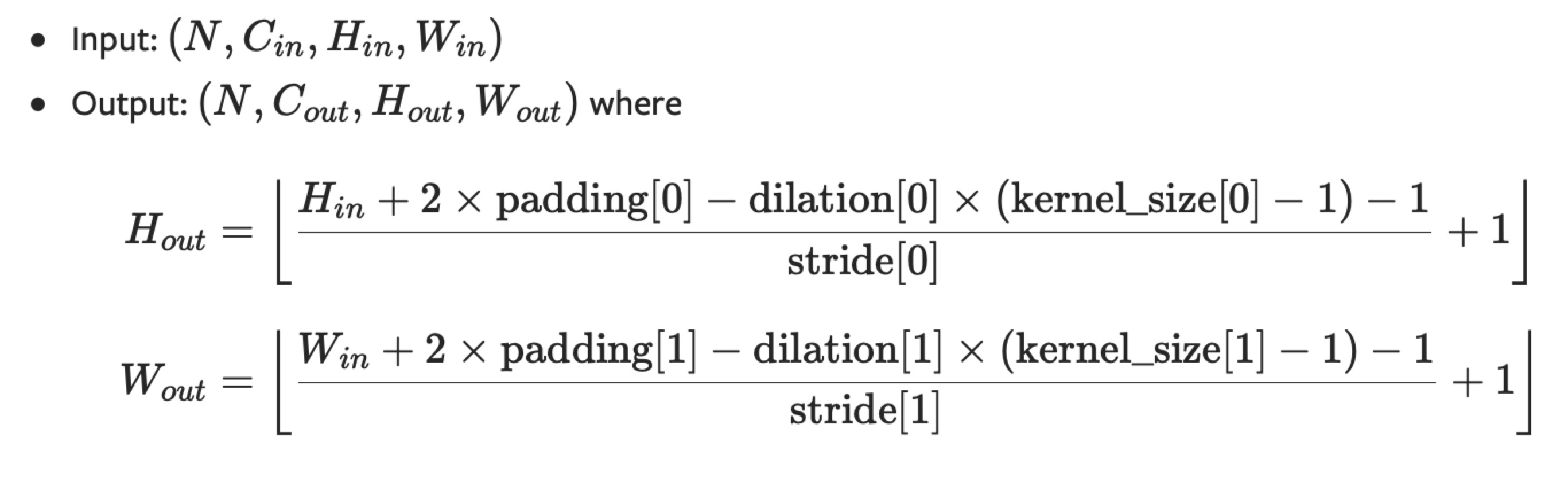weight(tensor) - 卷积的权重，大小是(out_channels, in_channels,kernel_size)
bias(tensor) - 卷积的偏置系数，大小是（out_channel

stride: 控制相关系数的计算步长
dilation: 用于控制内核点之间的距离
groups: 控制输入和输出之间的连接：

• group=1，输出是所有的输入的卷积；
• group=2，此时相当于有并排的两个卷积层，每个卷积层计算输入通道的一半，并且产生的输出是输出通道的一半，随后将这两个输出连接起来。

• 可以是一个int类型的数据，此时卷积height和width值相同;
• 也可以是一个tuple数组（包含来两个int类型的数据），第一个int数据表示height的数值，第二个int类型的数据表示width的数值

• in_channels(int) – 输入信号的通道数
• out_channels(int) – 卷积产生的通道数
• kerner_size(int or tuple) - 卷积核的大小
• stride(int or tuple,optional) - 卷积步长
• padding(int or tuple, optional) - 输入的每一条边补充padding= kernel - 1 - padding,即(kernel_size - 1)/2个0的层数，所以补充完高宽都增加(kernel_size - 1)
• output_padding(int or tuple, optional) - 在输出的每一个维度的一边补充0的层数，所以补充完高宽都增加padding，而不是2*padding，因为只补一边
• dilation(int or tuple, optional) – 卷积核元素之间的间距
• groups(int, optional) – 从输入通道到输出通道的阻塞连接数
• bias(bool, optional) - 如果bias=True，添加偏置

shape: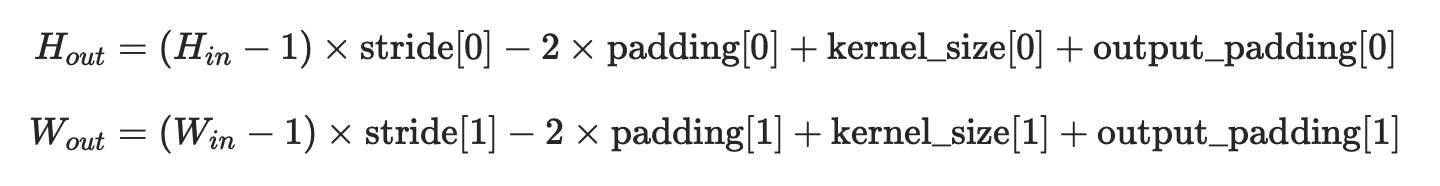- weight(tensor) - 卷积的权重，大小是(in_channels, in_channels,kernel_size)  ,从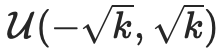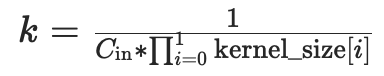中进行采样
- bias(tensor) - 卷积的偏置系数，大小是（out_channel ,从中进行采样

⚠️deconv只能做到还原输出大小到和卷积输入大小一样大，输出值和卷积输入有那么一点联系

import torch
from torch import nn
input = torch.randn(1,16,12,12)
h = downsample(input)
h.size()

torch.Size([1, 16, 6, 6])

output = upsample(h, output_size=input.size())
output.size()

torch.Size([1, 16, 12, 12])

output = upsample(h)
output.size()

torch.Size([1, 16, 12, 12])

upsample = nn.ConvTranspose2d(16,16,3,stride=2,padding=1,output_padding=1)

2）标准化层

### class torch.nn.BatchNorm2d(num_features, eps=1e-05, momentum=0.1, affine=True)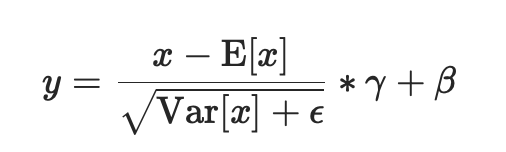• 先对输入进行归一化，E(x)为计算的均值，Var(x)为计算的方差
• 然后对归一化的结果进行缩放和平移，设置affine=True，即意味着weight(γ)和bias(β)将被使用

⚠️这个momentum参数不同于优化器optimizer类中使用的momentum参数和momentum的传统概念。从数学上讲，这里运行统计数据的更新规则是 :

• x是估计的数据
• xt是新的观察到的数据

xnew = (1-momentum) * x + momentum * xt

• num_features： C来自期待的输入大小(N,C,H,W)
• eps： 即上面式子中分母的ε ，为保证数值稳定性（分母不能趋近或取0）,给分母加上的值。默认为1e-5。
• momentum： 动态均值和动态方差所使用的动量。默认为0.1。
• affine： 一个布尔值，当设为true，给该层添加可学习的仿射变换参数，即γ与β。
• track_running_stats：一个布尔值，当设置为True时，该模块跟踪运行的平均值和方差，当设置为False时，该模块不跟踪此类统计数据，并且始终在train和eval模式中使用批处理统计数据。默认值:True

Shape：

import torch
from torch import nn

m = nn.BatchNorm2d(2,affine=True)
print(m.weight)
print(m.bias)

input = torch.randn(1,2,3,4)
print(input)
output = m(input)
print(output)
print(output.size())

Parameter containing:
Parameter containing:
tensor([[[[ 0.8316, -1.6250,  0.9072,  0.2746],
[ 0.4579, -0.2228,  0.4685,  1.2020],
[ 0.8648, -1.2116,  1.0224,  0.7295]],

[[ 0.4387, -0.8889, -0.8999, -0.2775],
[ 2.4837, -0.4111, -0.6032, -2.3912],
[ 0.5622, -0.0770, -0.0107, -0.6245]]]])
tensor([[[[ 0.3205, -1.1840,  0.3668, -0.0206],
[ 0.0916, -0.3252,  0.0982,  0.5474],
[ 0.3409, -0.9308,  0.4373,  0.2580]],

[[ 0.2664, -0.2666, -0.2710, -0.0211],
[ 1.0874, -0.0747, -0.1518, -0.8697],
[ 0.3160,  0.0594,  0.0860, -0.1604]]]],
torch.Size([1, 2, 3, 4])

import torch
from torch import nn

m = nn.BatchNorm2d(2,affine=False)
print(m.weight)
print(m.bias)

input = torch.randn(1,2,3,4)
print(input)
output = m(input)
print(output)
print(output.size())

None
None
tensor([[[[-1.5365,  0.2642,  1.0482,  2.0938],
[-0.0906,  1.8446,  0.7762,  1.2987],
[-2.4138, -0.5368, -1.2173,  0.2574]],

[[ 0.2518, -1.9633, -0.0487, -0.0317],
[-0.9511,  0.2488,  0.3887,  1.4182],
[-0.1422,  0.4096,  1.4740,  0.5241]]]])
tensor([[[[-1.2739,  0.0870,  0.6795,  1.4698],
[-0.1811,  1.2814,  0.4740,  0.8689],
[-1.9368, -0.5183, -1.0326,  0.0819]],

[[ 0.1353, -2.3571, -0.2028, -0.1837],
[-1.2182,  0.1320,  0.2894,  1.4478],
[-0.3080,  0.3129,  1.5106,  0.4417]]]])
torch.Size([1, 2, 3, 4])

3）池化层

### class torch.nn.MaxPool2d(kernel_size, stride=None, padding=0, dilation=1, return_indices=False, ceil_mode=False)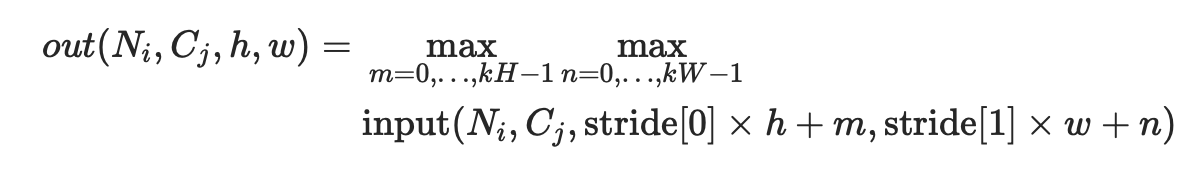dilation用于控制内核点之间的距离

• 可以是一个int类型的数据，此时卷积height和width值相同;
• 也可以是一个tuple数组（包含来两个int类型的数据），第一个int数据表示height的数值，tuple的第二个int类型的数据表示width的数值

• kernel_size(int or tuple) - max pooling的窗口大小
• stride(int or tuple, optional) - max pooling的窗口移动的步长。默认值是kernel_size
• padding(int or tuple, optional) - 输入的每一条边补充0的层数
• dilation(int or tuple, optional) – 一个控制窗口中元素步幅的参数
• return_indices - 如果等于True，会返回输出最大值的序号，对于上采样操作torch.nn.MaxUnpool2d会有帮助
• ceil_mode - 如果等于True，计算输出信号大小的时候，会使用向上取整ceil，代替默认的向下取整floor的操作

shape: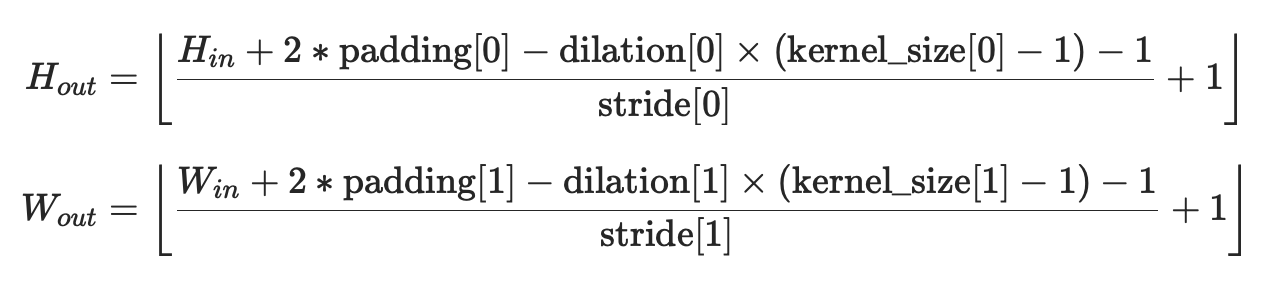• output_size: 输出信号的尺寸,可以用(H,W)表示H*W的输出，也可以使用单个数字H表示H*H大小的输出

input = torch.randn(1,64,8,9)
output = m(input)
output.shape

torch.Size([1, 64, 5, 7])

input = torch.randn(1,64,8,9)
output = m(input)
output.shape

torch.Size([1, 64, 7, 7])

4)非线形激活函数

class torch.nn.ReLU(inplace=False)

{ReLU}(x)= max(0, x)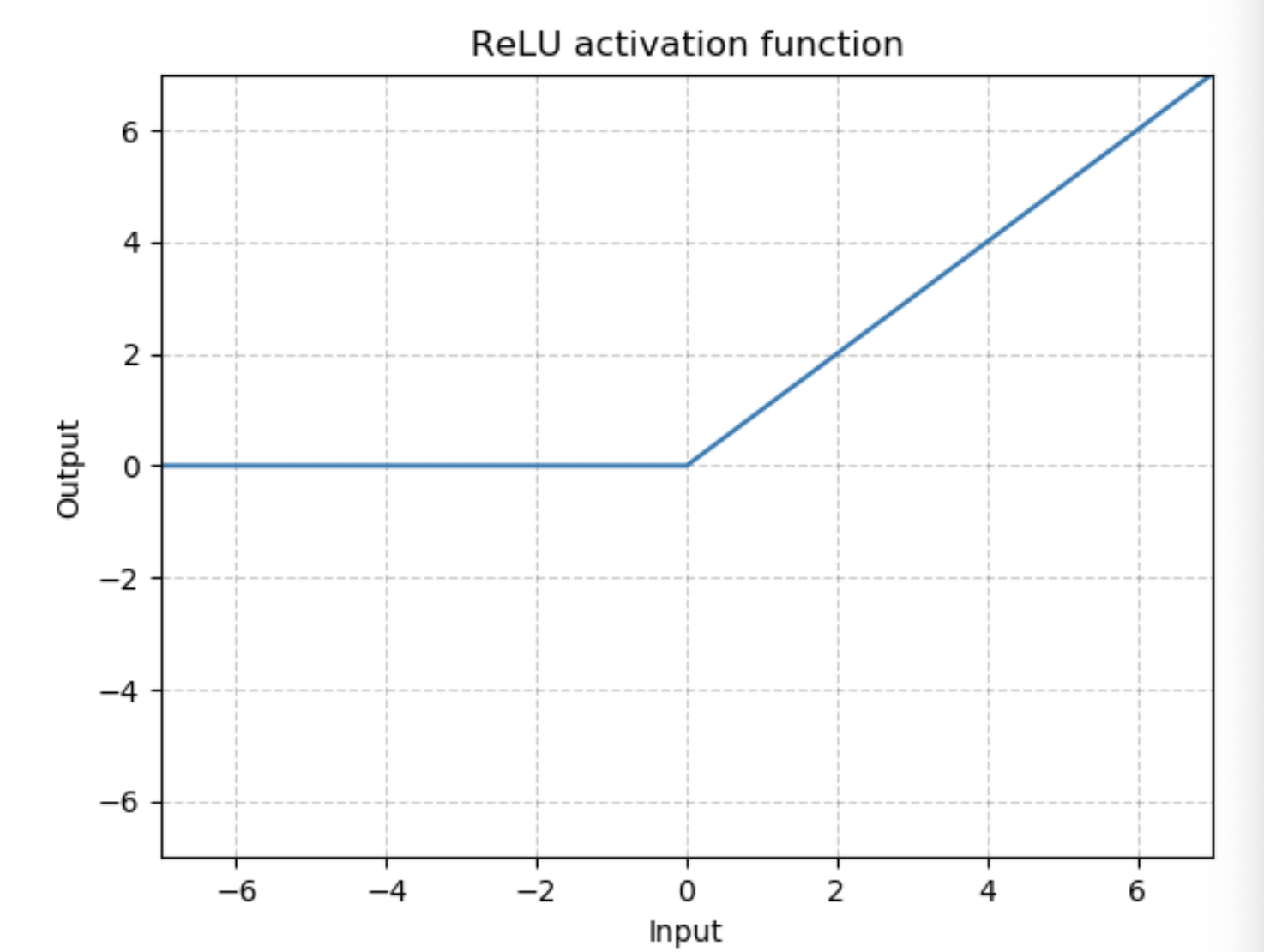shape：

• 输入：(N,*), *代表任意数目附加维度
• 输出：(N,*)，与输入拥有同样的形状

举例：

import torch
from torch import nn
m = nn.ReLU()
input = torch.randn(2)
output = m(input)
input, output

(tensor([-0.0468,  0.2225]), tensor([0.0000, 0.2225]))

class torch.nn.LeakyReLU(negative_slope=0.01, inplace=False)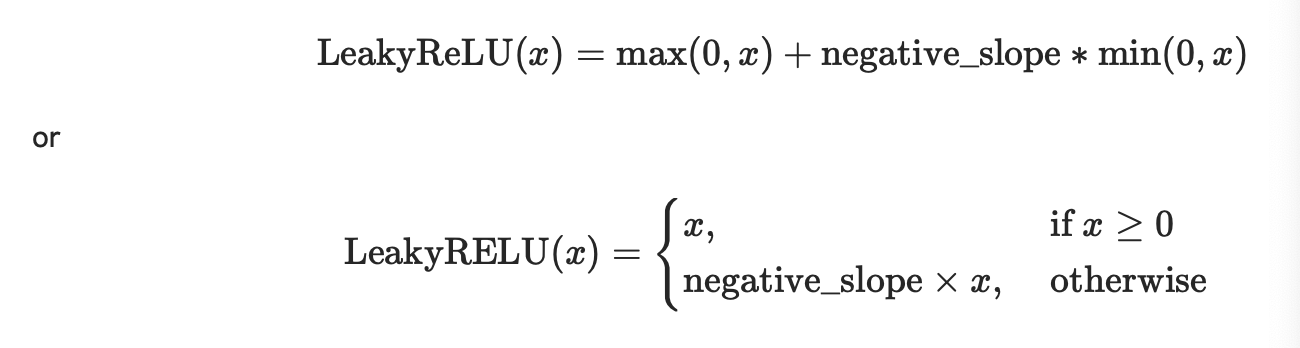• negative_slope：控制负斜率的角度，默认等于0.01
• inplace-选择是否进行原位运算，即x= x+1，默认为False

• 输入：(N,*), *代表任意数目附加维度
• 输出：(N,*)，与输入拥有同样的形状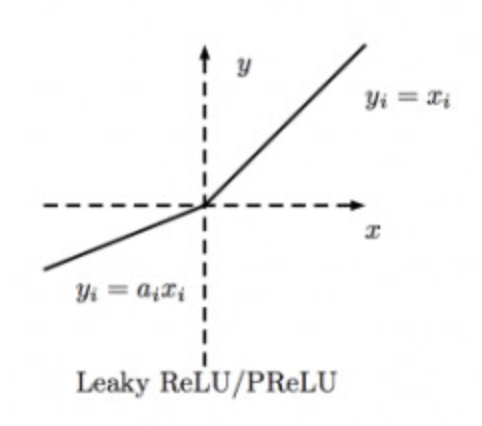m = nn.LeakyReLU(0.1)
input = torch.randn(2)
output = m(input)
input,output

(tensor([-1.3222,  0.8163]), tensor([-0.1322,  0.8163]))

class torch.nn.Sigmoid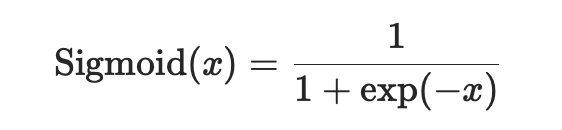• 输入：(N,*), *代表任意数目附加维度
• 输出：(N,*)，与输入拥有同样的形状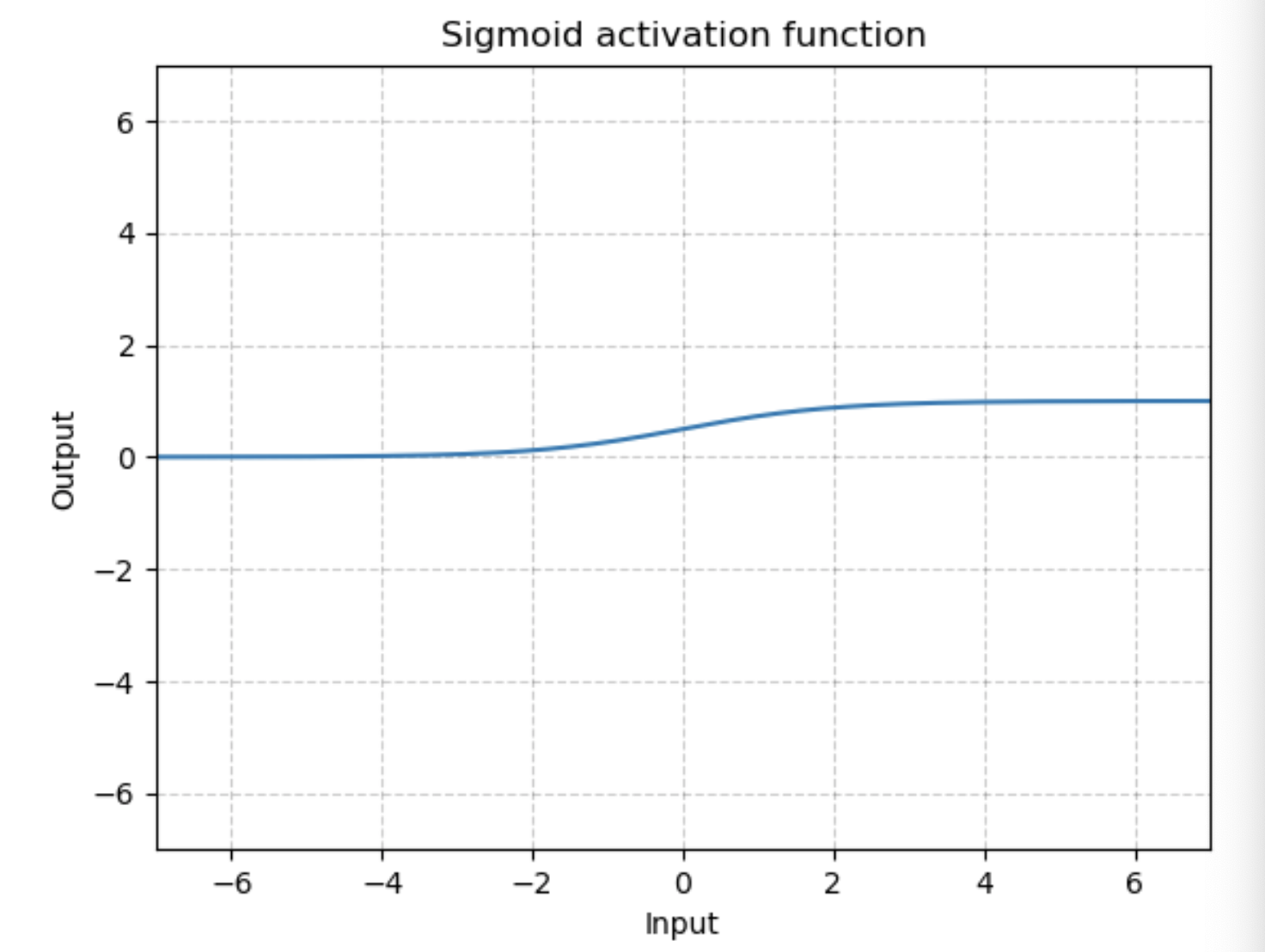m = nn.Sigmoid()
input = torch.randn(2)
output = m(input)
input, output

(tensor([-0.8425,  0.7383]), tensor([0.3010, 0.6766]))

class torch.nn.Tanh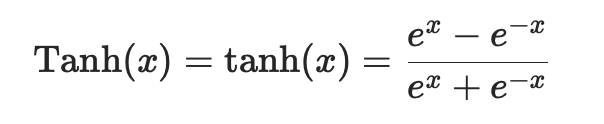• 输入：(N,*), *代表任意数目附加维度
• 输出：(N,*)，与输入拥有同样的形状

图为：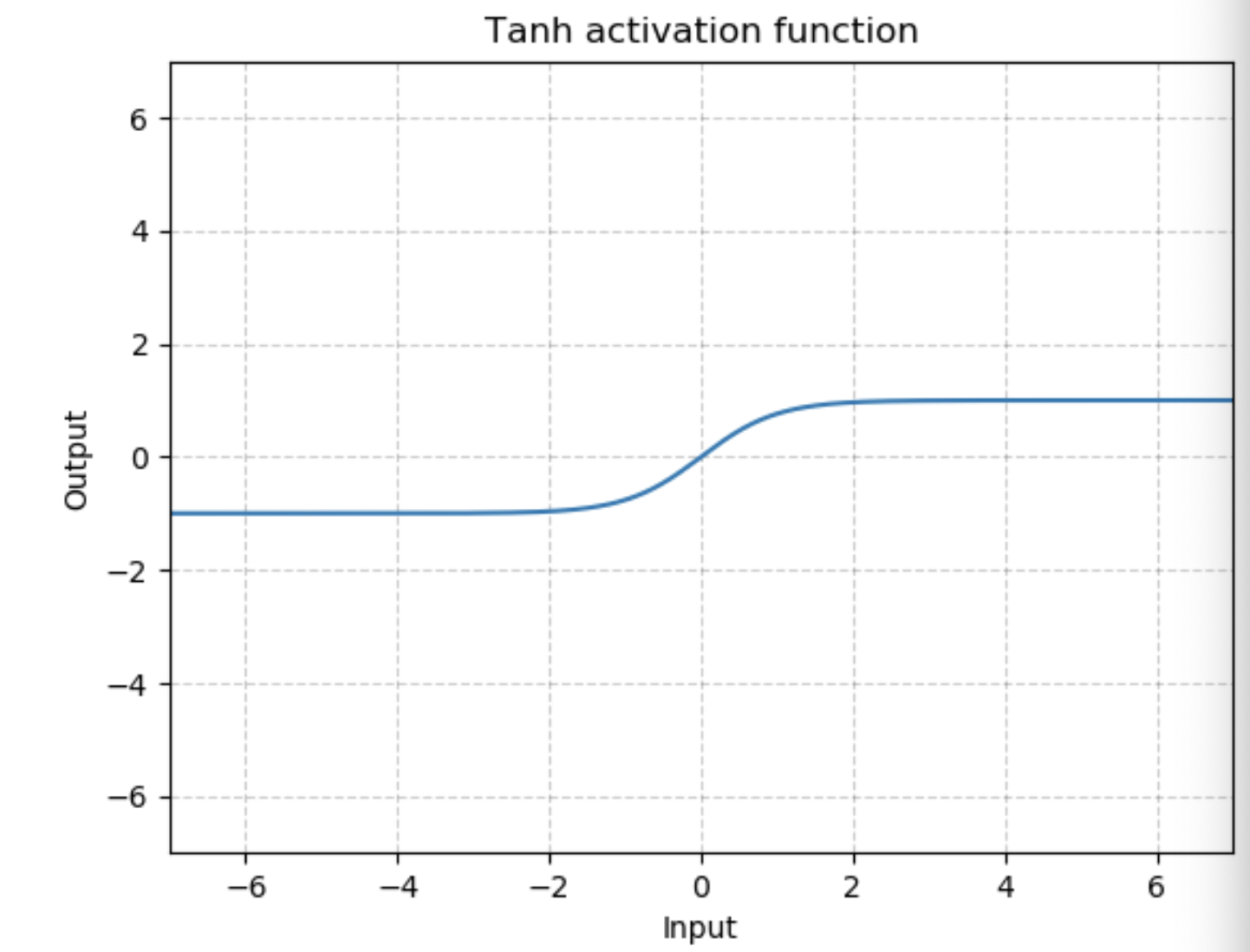m = nn.Tanh()
input = torch.randn(2)
output = m(input)
input, output

(tensor([-0.6246,  0.1523]), tensor([-0.5543,  0.1512]))

5）损失函数

### class torch.nn.BCELoss(weight=None, size_average=True, reduce=None, reduction='mean')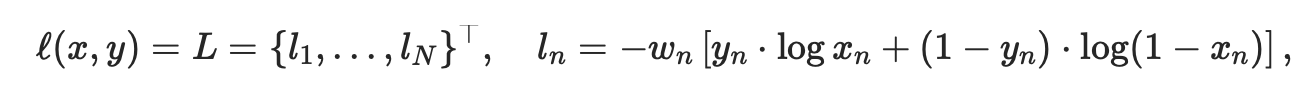N是批处理大小。如果reduce=True,则：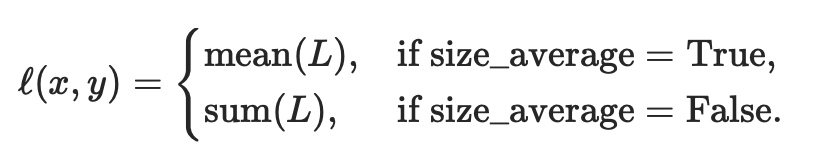这是用来测量误差error的重建，例如一个自动编码器。注意 0<=target[i]<=1。

• weight (Tensor,可选) – a manual rescaling weight given to the loss of each batch element. If given, has to be a Tensor of size “nbatch”.每批元素损失的手工重标权重。如果给定，则必须是一个大小为“nbatch”的张量。
• size_average (bool, 可选) – 弃用(见reduction参数)。默认情况下，设置为True，即对批处理中的每个损失元素进行平均。注意，对于某些损失，每个样本有多个元素。如果字段size_average设置为False，则对每个小批的损失求和。当reduce为False时，该参数被忽略。默认值:True
• reduce (bool,可选) – 弃用(见reduction参数)。默认情况下，设置为True，即根据size_average参数的值决定对每个小批的观察值是进行平均或求和。如果reduce为False，则返回每个批处理元素的损失，不进行平均和求和操作，即忽略size_average参数。默认值:True
• reduction (string,可选) – 指定要应用于输出的reduction操作:' none ' | 'mean' | ' sum '。“none”:表示不进行任何reduction，“mean”:输出的和除以输出中的元素数，即求平均值，“sum”:输出求和。注意:size_average和reduce正在被弃用，与此同时，指定这两个arg中的任何一个都将覆盖reduction参数。默认值:“mean”

形状：

• 输入：(N,*), *代表任意数目附加维度
• 目标：(N,*)，与输入拥有同样的形状
• 输出:标量scalar，即输出一个值。如果reduce为False,即不进行任何处理，则(N,*)，形状与输入相同。

举例：

m = nn.Sigmoid()
loss = nn.BCELoss()
target = torch.empty(3).random_(2)
output = loss(m(input), target)
output.backward()

input,target,output

(tensor([-0.8728,  0.3632, -0.0547], requires_grad=True),
tensor([1., 0., 0.]),
tensor(0.9264, grad_fn=<BinaryCrossEntropyBackward>))

input.grad

tensor([-0.2351,  0.1966,  0.1621])

posted @ 2019-04-28 15:58  慢行厚积  阅读(13390)  评论(0编辑  收藏